# Volume 26, 2020, Number 2

Volume 26Number 1 ▷ Number 2 ▷ Number 3Number 4

Bi-unitary multiperfect numbers, II
Original research paper. Pages 1—26
Pentti Haukkanen and Varanasi Sitaramaiah
Full paper (PDF, 258 Kb) | Abstract

A divisor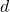of a positive integer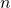is called a unitary divisor ifandis called a bi-unitary divisor ofif the greatest common unitary divisor ofand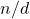is unity. The concept of a bi-unitary divisor is due to D. Surynarayana (1972). Let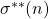denote the sum of the bi-unitary divisors of. A positive integeris called a bi-unitary multiperfect number if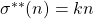for some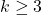. For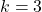we obtain the bi-unitary triperfect numbers.

Peter Hagis (1987) proved that there are no odd bi-unitary multiperfect numbers. The present paper is Part II in a series of papers on even bi-unitary multiperfect numbers. In the first part we found all bi-unitary triperfect numbers of the form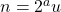, whereand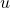is odd; the only one being. In this second part we find all bi-unitary triperfect numbers in the cases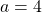and. Forthe only one is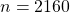, and forthey are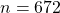,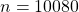,and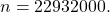Some modular considerations regarding odd perfect numbers
Original research paper. Pages 27—33
Jose Arnaldo Bebita Dris and Immanuel Tobias San Diego
Full paper (PDF, 143 Kb) | Abstract

Letbe an odd perfect number with special prime. In this article, we provide an alternative proof for the biconditional thatholds if and only if. We then give an application of this result to the case when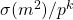is a square.

Restrictive factor and extension factor
Original research paper. Pages 34—46
József Sándor and Krassimir T. Atanassov
Full paper (PDF, 214 Kb) | Abstract

Restrictive factor and extension factor are two arithmetic functions, introduced by the authors. In the paper, some of their extensions are introduced and some of the basic properties of the newly defined functions are studied.

On multiplicative order of elements in finite fields based on cyclotomic polynomials
Original research paper. Pages 47—52
Roman Popovych
Full paper (PDF, 97 Kb) | Abstract

We obtain explicit lower bound on multiplicative orders of all elements in finite field extensions generated by a root of unity. The bound does not depend on any unknown constant. The result of Ahmadi, Shparlinski and Voloch  is a consequence of our main result.

Number of tuples with a given least common multiple
Original research paper. Pages 53—60
K. Siddharth Choudary and A. Satyanarayana Reddy
Full paper (PDF, 170 Kb) | Abstract

In this paper, for a given natural number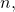we count the number of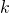-tuples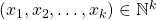with certain conditions such that lcmIn the process, we derived different arithmetic functions.

On the symmetrical second order hyperbolic quaternions sequences
Original research paper. Pages 61—70
Sure Köme and Cahit Köme
Full paper (PDF, 164 Kb) | Abstract

The purpose of this study is to obtain a new generalized quaternions sequences by using hyperbolic functions with second order recurrence sequences. First of all, we define the symmetrical second order hyperbolic sine and the symmetrical second order hyperbolic cosine quaternions. Then, we investigate norms and some relations between these type of quaternions. We also obtain generating functions, Binet formulas, Catalan’s identity, Cassini’s identity and d’Ocagne’s identity of second order hyperbolic quaternions sequences.

Higher-order identities for balancing numbers
Original research paper. Pages 71—84
Takao Komatsu
Full paper (PDF, 186 Kb) | Abstract

Let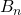be the-th balancing number. In this paper, we give some explicit expressions ofand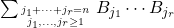. We also consider the convolution identities with binomial coefficients: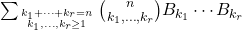.
This type can be generalized, so thatis a special case of the number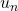, where(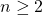) withand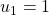.

Infinite series containing generalized harmonic functions
Original research paper. Pages 85—104
Kwang-Wu Chen and Yi-Hsuan Chen
Full paper (PDF, 287 Kb) | Abstract

We use Abel’s summation formula and the method of partial fraction decomposition to study infinite series involving generalized harmonic numbers of any positive integral order, with any positive integral power.

The linear combination of two polygonal numbers is a perfect square
Original research paper. Pages 105—115
Mei Jiang and Yangcheng Li
Full paper (PDF, 200 Kb) | Abstract

By the theory of Pell equation and congruence, we study the problem about the linear combination of two polygonal numbers is a perfect square. Let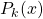denote the-th-gonal number. We show that if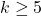,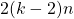is not a perfect square, and there is a positive integer solutionofsatisfyingthen the Diophantine equation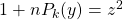has infinitely many positive integer solutions. Moreover, we give conditions aboutsuch that the Diophantine equation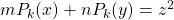has infinitely many positive integer solutions.

An alternative proof of Nyblom’s results and a generalisation
Original research paper. Pages 116—126
A. David Christopher
Full paper (PDF, 191 Kb) | Abstract

Letbe a positive integer andbe a non-negative integer. We define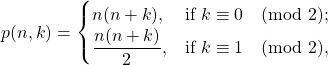and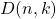to be the number of wayscan be expressed as a difference of two elements from the sequence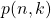. Nyblom found closed expressions forand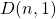in terms of some restricted number-of-divisors functions. Here we re-establish these two results of Nyblom in a relatively simple way. Along with the other interpretations for, an expression foris presented in terms of restricted form ofand. Also we consider another function due to Nyblom, denoted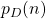, which counts the number of partitions ofwith parts in arithmetic progression having common difference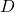. Nyblom and Evan found a simple expression for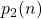and putin terms of a divisor-counting functions when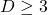. Here we re-establish Nyblom’s expression for, and find equinumerous expressions forwhen. Finally, we present the following generalised version of: given a set of positive integers say,, we denote by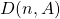, the number of wayscan be written as a difference of two elements from the set. And we expressin terms of partition enumerations when some restrictions are imposed upon the elements of. We close with the hint that, boundedness oftogether with the divergence of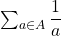disproves Erdős arithmetic progression conjecture.

Multifarious results for q-Hermite-based Frobenius-type Eulerian polynomials
Original research paper. Pages 127—141
Waseem Ahmad Khan, Idrees Ahmad Khan, Mehmet Acikgoz and Ugur Duran
Full paper (PDF, 220 Kb) | Abstract

In this paper, a new class of q-Hermite-based Frobenius-type Eulerian polynomials is introduced by means of generating function and series representation. Several fundamental formulas and recurrence relations for these polynomials are derived via different generating methods. Furthermore, diverse correlations including the q-Apostol-Bernoulli polynomials, the q-Apostol-Euler poynoomials, the q-Apostol-Genocchi polynomials and the q-Stirling numbers of the second kind are also established by means of the their generating functions.

Saalschütz’ theorem and Rising binomial coefficients – Type 2
Original research paper. Pages 142—147
A. G. Shannon
Full paper (PDF, 98 Kb) | Abstract

This paper extends some work of Leonard Carlitz on rising binomial coefficients and hypergeometric series in the context of a result of Louis Saalschütz which has animated further work in a number of branches of mathematics as well as physics.

A new explicit formula for the Bernoulli numbers in terms of the Stirling numbers of the second kind
Original research paper. Pages 148—151
Sumit Kumar Jha
Full paper (PDF, 129 Kb) | Abstract

Let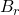denote the Bernoulli numbers, and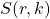denote the Stirling numbers of the second kind. We prove the following explicit formula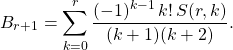To the best of our knowledge, the formula is new.

Infinite sums associated with certain Lucas sequences
Original research paper. Pages 152—158
S. G. Rayaguru and G. K. Panda
Full paper (PDF, 169 Kb) | Abstract

We obtain some closed form expressions for certain infinite sums related to Lucas and associated Lucas sequences. Some of our results generalize existing results concerning infinite sums involving Lucas sequences and others are variants of some existing results.

Infinite series involving Fibonacci numbers and the Riemann zeta function
Original research paper. Pages 159—166
Robert Frontczak
Full paper (PDF, 185 Kb) | Abstract

Two new closed forms for infinite series involving Fibonacci numbers and the Riemann zeta function are derived using standard methods from complex analysis. Also, expressions for the companion series with Lucas numbers are presented.

Circular-hyperbolic Fibonacci quaternions
Original research paper. Pages 167—176
Fügen Torunbalcı Aydın
Full paper (PDF, 194 Kb) | Abstract

In this paper, circular-hyperbolic Fibonacci numbers and quaternions are defined. Also, some algebraic properties of circular-hyperbolic Fibonacci numbers and quaternions which are connected with circular-hyperbolic numbers and Fibonacci numbers are investigated. Furthermore, Honsberger’s identity, the generating function, Binet’s formula, d’Ocagne’s identity, Cassini’s identity, and Catalan’s identity for these quaternions are given.

On r-circulant matrices with Horadam numbers having arithmetic indices
Original research paper. Pages 177—197
Aldous Cesar F. Bueno
Full paper (PDF, 238 Kb) | Abstract

We investigate an r-circulant matrix whose entries are Horadam numbers having arithmetic indices. We then solve for the eigenvalues, determinant, Euclidean norm and spectral norm of the matrix. Lastly, we present some special cases and some results on identities and divisibility.

On generalized Fibonacci quadratics
Original research paper. Pages 198—204
Neşe Ömür and Zehra Betül Gür
Full paper (PDF, 193 Kb) | Abstract

In this paper, we consider generalized
Fibonacci quadratics and give solutions of them under certain conditions.
For example, for odd numberunder condition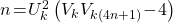, the equation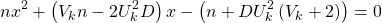has rational roots.

On generalized order-k modified Pell and Pell–Lucas numbers in terms of Fibonacci and Lucas numbers
Original research paper. Pages 205—212
Ahmet Daşdemir
Full paper (PDF, 345 Kb) | Abstract

This study shows that the generalized order-k Pell–Lucas and Modified Pell numbers can be expressed in terms of the well-known Fibonacci numbers. Certain n-square Hessenberg matrices with permanents equal to the Fibonacci numbers are defined. These Hessenberg matrices are then extended to super-diagonal (0,1,2)-matrices. In particular, the permanents of the super-diagonal matrices are shown to equal the components of the generalized order-k Pell–Lucas and Modified Pell numbers, and also their sums. In addition, two computer algorithms regarding our results are composed.

An identity for vertically aligned entries in Pascal’s triangle
Original research paper. Pages 213—221
Heidi Goodson
Full paper (PDF, 156 Kb) | Abstract

The classic way to write down Pascal’s triangle leads to entries in alternating rows being vertically aligned. In this paper, we prove a linear relation on vertically aligned entries in Pascal’s triangle. Furthermore, we give an application of this relation to morphisms between hyperelliptic curves.

This issue of the International Journal “Notes on Number Theory and Discrete Mathematics” is published with the financial support of the Bulgarian National Science Fund, Grant Ref. No. KP-06-NP1-15/2019.

Volume 26Number 1 ▷ Number 2 ▷ Number 3Number 4

Comments are closed.